• 已经处理退化的多边形裁剪算法 //编译环境：Visual C++ 6.0，EasyX_20190219(beta) #include<graphics.h> #include<conio.h> #include<iostream> #define max 30 using namespace std; //设置...
已经处理退化边的多边形裁剪算法
//编译环境：Visual C++ 6.0，EasyX_20190219(beta)
#include<graphics.h>
#include<conio.h>
#include<iostream>
#define max 30
using namespace std;

//设置裁剪框的大小和位置,裁剪多边形顶点和顶点数，以全局变量给出
double xl=5,xr=140,yt=190,yb=74;
int inlength=9;
POINT Vertex[]={ {110,84},{160,94},{90,169},{90,94},{70,90},{50,230}, {165,230},{175,89},{163,54} };
//求多边形的一条边sp和裁剪边point0 point1的交点
void Intersect(POINT S,POINT P,POINT point0,POINT point1,POINT &I)
{
if(point0.y==point1.y)//水平裁剪边
{
I.y=point0.y;
I.x=S.x+(point0.y-S.y)*(P.x-S.x)/(P.y-S.y);
}
else//竖直裁剪边
{
I.x=point0.x;
I.y=S.y+(point0.x-S.x)*(P.y-S.y)/(P.x-S.x);
}
}

//测试顶点与裁剪边的内外关系
bool Inside(POINT text,POINT point0,POINT point1)
{
if(point1.x>point0.x){//裁剪边为窗口的下边
if(text.y>=point0.y)
return true;}

else if(point1.x<point0.x){//裁剪边为窗口的上边
if(text.y<=point0.y)
return true;}

else if(point1.y>point0.y){//裁剪边为窗口的右边
if(text.x<=point0.x)
return true;}

else if(point1.y<point0.y){//裁剪边为窗口的左边
if(text.x>=point0.x)
return true;}

return false;
}

//将新的定点加入结果多边形顶点表
void Output(POINT newpoint,int &length,POINT outps[])
{
outps[length].x=newpoint.x;
outps[length].y=newpoint.y;
length++;
}

//裁剪算法
void SutherlandHodgmanPolygonClip(int inlength,POINT inpoints[],int &outlength,POINT outpoints[],POINT point0,POINT point1)
{
POINT S,P,I;
int j;
outlength=0;
S=inpoints[inlength-1];
for(j=0;j<inlength;j++)
{
P=inpoints[j];
if(Inside(P,point0,point1))
{
if(Inside(S,point0,point1))
{Output(P,outlength,outpoints);}
else
{
Intersect(S,P,point0,point1,I);
Output(I,outlength,outpoints);
Output(P,outlength,outpoints);}}
else if(Inside(S,point0,point1))
{
Intersect(S,P,point0,point1,I);
Output(I,outlength,outpoints);}
S=P;}
}

int main()
{
POINT Edge[]={ {xr,yb},{xr,yt},{xl,yt},{xl,yb} };
initgraph(1000,900);
polygon(Edge,4);
setcolor(RED);
polygon(Vertex,inlength);
int i;
POINT OutPts1[max],OutPts2[max],OutPts3[max],OutPts4[max];
int length1,length2,length3,length4;
SutherlandHodgmanPolygonClip(inlength,Vertex,length1,OutPts1,Edge,Edge);//右边窗口裁剪边
SutherlandHodgmanPolygonClip(length1,OutPts1,length2,OutPts2,Edge,Edge);//下边窗口裁剪边
SutherlandHodgmanPolygonClip(length2,OutPts2,length3,OutPts3,Edge,Edge);//左边窗口裁剪边
SutherlandHodgmanPolygonClip(length3,OutPts3,length4,OutPts4,Edge,Edge);//上边窗口裁剪边
MOUSEMSG m;
while(true)
{
m=GetMouseMsg();
switch(m.uMsg)
{
case WM_LBUTTONDOWN:
cleardevice();
setcolor(RED);
polygon(Edge,4);
setcolor(YELLOW);
polygon(OutPts4,length4);
for(i=0;i<length4;i++)
{OutPts4[i].x+=300;}
polygon(OutPts4,length4);
break;
case WM_RBUTTONDOWN:
return 0;
}
}
_getch();
closegraph();
return 0;
}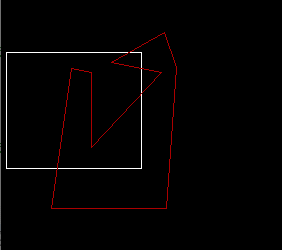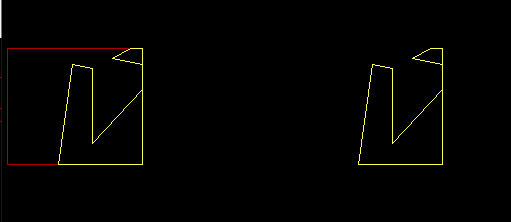以上是按照右下左上的顺序进行裁剪的，当然读者可以按照自己的习惯进行调整。


展开全文多边形裁剪
• （1）算法设计原理 （2）程序关键代码 void S_L_Line(int inlen,POINT inpoints[],int &outlen,POINT outpoints[],POINT point0,POINT point1) { outlen=0; POINT P2,P1,I; int j; P2=inpoints[inlen-1]; /...
（1）算法设计原理（2）程序关键代码
void S_L_Line(int inlen,POINT inpoints[],int &outlen,POINT outpoints[],POINT point0,POINT point1)
{
outlen=0;
POINT P2,P1,I;
int j;

P2=inpoints[inlen-1];  //p1=p时，p2=p[length-1]
for(j=0;j<inlen;j++)
{
P1=inpoints[j];
if(Inside(P1,point0,point1))    //当P1在边界内
{
if(Inside(P2,point0,point1))  //当P2在边界内
{Output(P1,outlen,outpoints);}
else                             //当P2不在边界内
{
jiaodian(P2,P1,point0,point1,I);
Output(I,outlen,outpoints);
Output(P1,outlen,outpoints);}}
else if(Inside(P2,point0,point1))   //当P1在边界内且P2在边界内
{
jiaodian(P2,P1,point0,point1,I);
Output(I,outlen,outpoints);}
P2=P1;}
}

（3）运行结果截屏（对数据输入的说明）（4）算法的不足之处
没有实现退化边处理
完整代码：
#include<graphics.h>
#include<conio.h>
#include<iostream>
#define max 30
using namespace std;

double xl=5,xr=140,yt=190,yb=75;
int inlen=9;
POINT Vertex[]={ {110,80},{160,90},{90,170},{90,95},{70,90},{50,230}, {165,230},{175,90},{160,50} };

//求多边形的边sp和边point0 point1的交点
void jiaodian(POINT S,POINT P,POINT point0,POINT point1,POINT &I)
{
if(point0.y==point1.y)   //水平裁剪边
{
I.y=point0.y;
I.x=S.x+(point0.y-S.y)*(P.x-S.x)/(P.y-S.y);  //计算交点
}
else
{
I.x=point0.x;
I.y=S.y+(point0.x-S.x)*(P.y-S.y)/(P.x-S.x);
}
}

//顶点与裁剪边的内外关系
bool Inside(POINT text,POINT point0,POINT point1)
{
if(point1.x>point0.x)
{
//下边
if(text.y>=point0.y)
return true;
}
else if(point1.x<point0.x)
{
//上边
if(text.y<=point0.y)
return true;
}

else if(point1.y>point0.y)
{
//裁剪边为窗口的右边
if(text.x<=point0.x)
return true;
}

else if(point1.y<point0.y)
{
//裁剪边为窗口的左边
if(text.x>=point0.x)
return true;
}
return false;
}

//将点加入多边形点集
void Output(POINT newpoint,int &len,POINT outps[])
{
outps[len].x=newpoint.x;
outps[len].y=newpoint.y;
len++;
}

void S_L_Line(int inlen,POINT inpoints[],int &outlen,POINT outpoints[],POINT point0,POINT point1)
{
outlen=0;
POINT P2,P1,I;
int j;

P2=inpoints[inlen-1];  //p1=p时，p2=p[length-1]
for(j=0;j<inlen;j++)
{
P1=inpoints[j];
if(Inside(P1,point0,point1))    //当P1在边界内
{
if(Inside(P2,point0,point1))  //当P2在边界内
{Output(P1,outlen,outpoints);}
else                             //当P2不在边界内
{
jiaodian(P2,P1,point0,point1,I);
Output(I,outlen,outpoints);
Output(P1,outlen,outpoints);}}
else if(Inside(P2,point0,point1))   //当P1在边界内且P2在边界内
{
jiaodian(P2,P1,point0,point1,I);
Output(I,outlen,outpoints);}
P2=P1;}
}

int main()
{
POINT E[]={ {xr,yb},{xr,yt},{xl,yt},{xl,yb} };
initgraph(1000,900);
polygon(E,4);
setcolor(RED);
polygon(Vertex,inlen);
int i;
POINT Opoint1[max],Opoint2[max],Opoint3[max],Opoint4[max];
int len1,len2,len3,len4;
S_L_Line(inlen,Vertex,len1,Opoint1,E,E);//右边
S_L_Line(len1,Opoint1,len2,Opoint2,E,E);//新的多边形点集进行下边的裁剪
S_L_Line(len2,Opoint2,len3,Opoint3,E,E);//左边
S_L_Line(len3,Opoint3,len4,Opoint4,E,E);//上边
MOUSEMSG m;
while(true)
{
m=GetMouseMsg();
switch(m.uMsg)
{
case WM_LBUTTONDOWN:
cleardevice();
setcolor(RED);
polygon(E,4);
setcolor(YELLOW);
polygon(Opoint4,len4);
break;
case WM_RBUTTONDOWN:
return 0;
}
}
_getch();
closegraph();
return 0;
}




展开全文• 逐边裁剪算法思想
多边形是由若干线段围成的封闭图形，使用长方形裁剪多边形得到的结果应该仍然是一个多边形，一个封闭的图形
之前我们学习了Liang-Barsky直线裁剪算法，但是如果仅重复的利用直线段的裁剪方法进行裁剪，很可能无法得到期望的图形结果，无法得到一个封闭的多边形
采用逐边裁剪算法:
依次使用窗口的四条边框直线对多边形进行分布裁剪，先用一条边框直线对整个多边形进行裁剪，得到一个或者若干个新的多边形，再用第二条边框直线对新产生的多边形进行裁剪…依次类推知道四条边框直线都裁剪完，整个多边形的裁剪过程结束
步骤：

把待裁剪多边形的各个顶点按照一定的方向有次序的组成有序的顶点序列（p1,p2,pn），相继连接相邻两个顶点（p1p2,p2p3,p3p4,pnp1)即为组成多边形的n条边。该顶点序列就是待处理的输入量
对输入的顶点序列进行处理，结果是产生一组新的顶点序列，然后输出该组新的顶点序列，该新的顶点序列表示了由M条边组成的新的多边形
把输入的顶点序列作为新的输入量，再次输入进行处理，这样处理三次

如何处理：

对于给定的顶点序列，依次检验顶点序列中每个顶点pi (i=1,2,n) ，处于裁剪边框可见侧的顶点被放入新的顶点序列中，处于裁剪边框不可见侧的顶点被删除
还要检测pi和他的前一个顶点pi-1点是否处于裁剪边框的同侧（认为p1的前一个顶点是pn），如果不再同一侧的话，要求出裁剪边框与直线段pipi-1的交点并把该交点作为新的顶点列入到新顶点序列中输出
我们设多边形的任意一条边起点为s，终点为e。给定的矩形有可见侧和不可见侧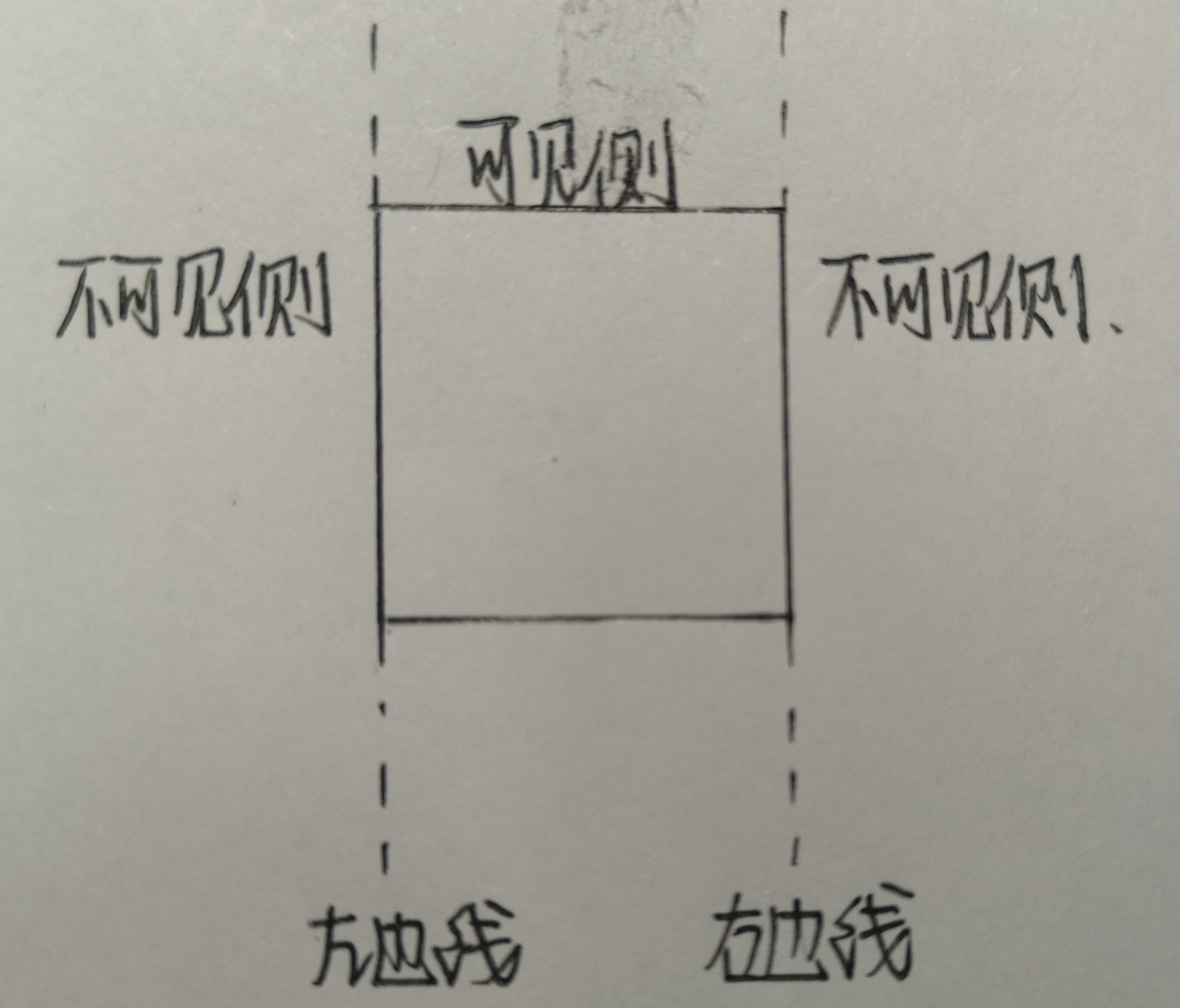则线段SE和裁剪线有四种位置关系：
端点SE都在不可见侧，则没有输出；SE都在可见侧，输出端点E；端点S在可见侧，端点E在不可见侧，则输出线段SE与裁剪线的交点C；端点S在不可见侧，端点E在可见侧，则输出线段SE与裁剪线的交点C和端点E

#include<GL/glut.h>
#include<iostream>
#include<algorithm>
#include<vector>
#include<map>
#include<time.h>
using namespace std;
const int window_width = 800, window_height = 600;
char op;
struct point
{
float x, y;
point() {}
point(float tempx, float tempy)
:x(tempx), y(tempy) {}
bool operator < (const point& a)const
{
return x < a.x;
}
};
struct EDGE
{
float bx, by, ex, ey;
EDGE() {}
EDGE(float bxx, float byy, float exx, float eyy)
:bx(bxx), by(byy), ex(exx), ey(eyy) {}
};
map<float, map<float, int> > vis;
vector<point> input_vertice;
vector<point> output_vertice;
float intersect_point_color = { 1,0,0 };
float polygon_point_color = { 0,0,1 };
EDGE left(200, 500, 200, 200);
EDGE bottom(200, 200, 500, 200);
EDGE right(500, 200, 500, 500);
EDGE top(500, 500, 200, 500);
void draw_rec()
{
glColor3f(1.0, 1.0, 0.0);
glLineWidth(2.0f);
glBegin(GL_LINES);
glVertex2f(::left.bx, ::left.by); glVertex2f(::left.ex, ::left.ey);
glVertex2f(bottom.bx, bottom.by); glVertex2f(bottom.ex, bottom.ey);
glVertex2f(::right.bx, ::right.by); glVertex2f(::right.ex, ::right.ey);
glVertex2f(top.bx, top.by); glVertex2f(top.ex, top.ey);
glEnd();
glFlush();
}
bool inside(point& pt, EDGE ClipBoundary)//判断点是否可见
{
if (ClipBoundary.ex > ClipBoundary.bx)
{
if (pt.y >= ClipBoundary.by)//裁剪边为窗口下边沿
return true;
}
else if (ClipBoundary.ex < ClipBoundary.bx)
{
if (pt.y <= ClipBoundary.by)//裁剪边为窗口上边沿
return true;
}
else if (ClipBoundary.ey > ClipBoundary.by)//裁剪边为窗口右边沿
{
if (pt.x <= ClipBoundary.bx)
return true;
}
else if (ClipBoundary.ey < ClipBoundary.by)//裁剪边为窗口左边沿
{
if (pt.x >= ClipBoundary.bx)
return true;
}
return false;
}
void intersect(point& s, point& p, EDGE ClipBoundary, point& intersect_pt)//直线段SP和边界求交，返回交点
{
if (ClipBoundary.by == ClipBoundary.ey)//水平裁剪边界
{
intersect_pt.y = ClipBoundary.by;
//x=起点的横坐标+dy/sp斜率
intersect_pt.x = s.x + (ClipBoundary.by - s.y) * (p.x - s.x) / (p.y - s.y);
}
else//垂直裁剪边界
{
intersect_pt.x = ClipBoundary.bx;
intersect_pt.y = s.y + (ClipBoundary.bx - s.x) * (p.y - s.y) / (p.x - s.x);
}
}
void draw_a_point(float x, float y, float color[])
{
glPointSize(5.0f);
glBegin(GL_POINTS);
glColor3fv(color);
glVertex2f(x, y);
glEnd();
glFlush();
}
void SutherlandHodgmanClip(EDGE ClipBoundary)
{
point s, p, ip;
output_vertice.clear();
s = input_vertice[input_vertice.size() - 1];//首尾
for (int j = 0; j < input_vertice.size(); j++)
{
p = input_vertice[j];
cout << "s" << s.x << "  " << s.y << endl;
cout << "p" << p.x << "  " << p.y << endl;
if (inside(p, ClipBoundary))//p在内
{
if (inside(s, ClipBoundary))//sp都在窗口内
{
//output(p);
output_vertice.push_back(p);
}
else//p在里面 s不在
{
intersect(s, p, ClipBoundary, ip);
output_vertice.push_back(ip);
output_vertice.push_back(p);
}
}
else//s在外面
{
if (inside(s, ClipBoundary))//s在窗口内p在窗口外
{
intersect(s, p, ClipBoundary, ip);
//output(ip);
output_vertice.push_back(ip);
}
//sp都在外面则无输出
}
s = p;
}
input_vertice = output_vertice;//这次的输出作为下一次的输入，input_vertice和output_vertice是全局变量
}
void draw_line_point(float x, float y)
{
vis[x][y]++;
glPointSize(2.5f);
glBegin(GL_POINTS);
if (vis[x][y] % 2 == 0)//访问偶数次，涂白色
{
glColor3f(1, 1, 1);
glVertex2f(x, y);
}
else
{
glColor3f(1, 0, 0);//奇数次，涂红色
glVertex2f(x, y);
}
glEnd();
glFlush();
}
void display() {
glClear(GL_COLOR_BUFFER_BIT);
glColor3f(1.0f, 0.0f, 0.0f);
glFlush();
}
void mymouse(int button, int state, int x, int y)
{
glClearColor(1, 1, 1, 1);
//bool flag = 0;
if (button == GLUT_LEFT_BUTTON && state == GLUT_DOWN)
{
if (op == 32)
{
glClear(GL_COLOR_BUFFER_BIT);
draw_rec();
input_vertice.clear();
op = 'a';
}
draw_a_point(x, window_height - y, polygon_point_color);
point p(x, window_height - y);
input_vertice.push_back(p);
cout << "多边形顶点" << input_vertice.size() << ":(" << x << ", " << window_height - y << ")" << endl;
}
if (button == GLUT_RIGHT_BUTTON && state == GLUT_DOWN)
{
glLineWidth(2.0f);
glBegin(GL_LINES);
glColor3fv(polygon_point_color);
for (int i = 0; i < input_vertice.size(); i++)
{
if (i == input_vertice.size() - 1)
{
glVertex2f(input_vertice.x, input_vertice.y);
glVertex2f(input_vertice[i].x, input_vertice[i].y);
}
else
{
glVertex2f(input_vertice[i].x, input_vertice[i].y);
glVertex2f(input_vertice[i + 1].x, input_vertice[i + 1].y);
}
}
glEnd();
glFlush();
}
}
void keyboard(unsigned char key, int x, int y)
{
if (key == 32)
{
op = 32;
SutherlandHodgmanClip(::left);
SutherlandHodgmanClip(bottom);
SutherlandHodgmanClip(::right);
SutherlandHodgmanClip(top);
glLineWidth(4.0f);
glBegin(GL_LINES);
glColor3fv(intersect_point_color);
for (int i = 0; i < output_vertice.size(); i++)
{
if (i == output_vertice.size() - 1)
{
glVertex2f(output_vertice.x, output_vertice.y);
glVertex2f(output_vertice[i].x, output_vertice[i].y);
}
else
{
glVertex2f(output_vertice[i].x, output_vertice[i].y);
glVertex2f(output_vertice[i + 1].x, output_vertice[i + 1].y);
}
}
glEnd();
glFlush();
}
}
int main(int argc, char* argv[])
{
glutInit(&argc, argv);
glutInitDisplayMode(GLUT_SINGLE | GLUT_RED);
glutInitWindowPosition(50, 50);
glutInitWindowSize(window_width, window_height);
glutCreateWindow("固定矩形窗口裁剪交互式多边形");
cout << "左键画点\n右键连接成窗口\n按空格裁剪\n" << endl;
glMatrixMode(GL_PROJECTION);
gluOrtho2D(0, window_width, 0, window_height);
glClearColor(1, 1, 1, 1);
glClear(GL_COLOR_BUFFER_BIT);
draw_rec();
glutMouseFunc(mymouse);
glutKeyboardFunc(keyboard);
glutDisplayFunc(&display);
glutMainLoop();
return 0;
}


展开全文• 图形学算法总结 文章目录图形学算法总结直线生成算法数值微分法（DDA）中点画线法Bresenham算法圆弧生成算法中点Bresenham...Barskey算法多边形逐边裁剪算法双边裁剪算法消隐深度缓存算法（Z_Buffer算法）扫描线算法多
图形学算法总结
文章目录图形学算法总结直线生成算法数值微分法（DDA）中点画线法Bresenham算法圆弧生成算法中点Bresenham画圆法多边形填充算法逐点判断法1）射线法2）累计角度法扫描线算法(YX)改进的扫描线算法（Y-X）边缘填充算法区域种子填充算法1）深度递归的种子填充算法(漫水法）2）扫描线种子填充算法反走样简单区域采样（非加权区域采样）加权区域采样直线和多边形裁剪编码裁剪中点分割裁剪梁友栋-Barskey算法多边形逐边裁剪算法双边裁剪算法消隐深度缓存算法（Z_Buffer算法）扫描线算法多边形区域排序算法曲线曲面Hermite曲线Bezier曲线B样条曲线图形变换平移变换比例变换旋转变换对称变换错切变换投影变换正交平行投影斜交投影透视投影
直线生成算法
数值微分法（DDA）
$y = kx + b$
x每增加1，y增量为k

|k|要小于1，大于则互换x，y
原因：防止画出来的线太稀疏象限
|dx|>|dy|?
D  x
D  y

1a
是
1
k

1b
否
1/k
1

2a
是
-1
k

2b
否
-1/k
1

3a
是
-1
-k

3b
否
-1/k
-1

4a
是
1
-k

4b
否
1/k
-1

中点画线法$y = ax + by +c$

其中，$a=y_0-y_1，b=x_1-x_0$

我们每次都把中点(x+1,y+0.5)带入方程，记结果为d，与0比较

d≥0 中点在直线上方，取$（x_i＋1，y_i）$

$d_{i+1}=d_i+a$

d<0 中点在直线下方，取$（x_i＋1，y_i＋1）$

$d_{i+1}=d_i+a+b$

其中，初始值$d_0 = a+0.5b$

可以用 2d 代替 d 来摆脱小数，提高效率。Bresenham算法我们计算直线的斜率 $k=\frac{dy}{dx}$
和DDA一样，x每增加1，y增加k
于是，我们可以这样算：
$d_0=0$   $d_{i+1} = d_i+k$

d≥0.5 取$（x_i＋1，y_i＋1）$
d<0.5 取$（x_i＋1，y_i）$

当 d≥1 的时候 $d = d-1$

当然，为了便于计算，可令 $e = d-0.5$
$e_0=-0.5$   $e_{i+1} = e_i+k$

e≥0 取$（x_i＋1，y_i＋1）$
e<0 取$（x_i＋1，y_i）$

当 e≥0 的时候 $e = e-1$圆弧生成算法
中点Bresenham画圆法$d = x^2+y^2-R^2$
与前面类似 $d_0=1.25-R$

d≥0  取$(x_i＋1，y_i-1)$

$d_{i+1}=d_i+2(x_p-y_p)+5$

d<0  取$(x_i＋1，y_i)$

$d_{i+1}=d_i++2x_p+3$

为方便计算，使用e=d-0.25代替d

多边形填充算法
逐点判断法
1）射线法
作射线求交点各数k

k为奇数 点在多边形内
k为偶数 点在多边形外

特殊情况：2）累计角度法与各顶点连线，形成有向角$\theta_i$，然后作累加

结果为0 点在多边形外
结果为±2$\pi$ 点在多边形内

扫描线算法(YX)举个例子，6号扫描线与多边形交点并按x值递增排序为A,B,C,D
然后我们就开始配对，AB一对，CD一堆，并给AB和CD这两个线段填色。
依此类推，就能给多边形填完色。

改进的扫描线算法（Y-X）活性边表：$x$ $△x$ $y_{max}$边表桶：特殊情况：

水平边扔掉

X为小数(a)交点位于左边上，向右取整
(b)交点位于右边上，向左取整

(x,e)落在像素上(a)(x,e)位于左边上，属于多边形
(b)(x,e)位于右边上，不属于多边形

交点位多边形的顶点（下闭上开）应该是指经过P1的点

(a) 算作1个交点
(b) 算作1个交点
(d) 算作0个交点

边缘填充算法
对多边形上每一条非水平边上的每个象素开始向右求余改进后有栅栏填充算法和边界标志算法

区域种子填充算法1）深度递归的种子填充算法(漫水法）

种子入栈

栈非空，转3；栈空，结束

栈顶元素出栈，如果它未填充则填充，然后找其他方向未填充的点，将其入栈，找完所有方向后（4联通就是4个方向，8联通就是8个方向），转2假设方向是上下左右，那么顺序就是

栈：H
填色：H 栈： I F J
填色：J 栈：I F K N
填色：N 栈：I F K M
填色：M 栈：I F K L
填色：L 栈：I F K
填色：K 栈：I F
填色：F 栈：I D G
填色：G 栈：I D E
填色：E 栈：I D
填色：D 栈：I C
填色：C 栈：I B
填色：B 栈：I A
填色：A 栈：I
填色：I

2）扫描线种子填充算法
其实就是种子填充的改进版，减少了递归次数
扫描线种子填充算法

初始化：堆栈置空。将种子点（x，y）入栈。

出栈：若栈空则结束。否则取栈顶元素（x，y），以y作为当前扫描线。

填充并确定种子点所在区段：从种子点（x，y）出发，沿当前扫描线向左、右两个方向填充，直到非内部。分别标记区段的左、右端点坐标为xl和xr。

并确定新的种子点：在区间[xl，xr]中检查与当前扫描线y上、下相邻的两条扫描线上的象素。若存在非边界、未填充的象素，则把每一区间的最右象素作为种子点压入堆栈，返回第（2）步。填充DFHJM
上下搜寻

DFHJM任意一个往下走填充EDIK
N往上走填充M
D往上走填充C

上下搜寻

M往上走填充L
C往上走填充B

上下搜寻

B往上走填充A

反走样

有两个方式，一个是提高分辨率，另一个是改进算法，这里当然讲算法喽

简单区域采样（非加权区域采样）
根据面积改变亮度达到反走样效果面积计算（m是斜率，像素宽为1）

情况(1)(5)阴影面积为：$\frac{D^2}{2m}$
情况(2)(4)阴影面积为：$D - \frac{m}{2}$
情况(3)阴影面积为：$1-\frac{D^2}{m}$

上述阴影面积是介于0-1之间的正数，用它乘以象素的最大灰度值，再取整，即可得到象素的显示灰度值。

加权区域采样
其实就是在非加权区域采样的基础上加了个权值来表示与中心距离，然后计算灰度的时候要考虑这个权值。直线和多边形裁剪
编码裁剪
$D_3D_2D_1D_0$分别表示是否上于上边界，是否下于下边界，是否右于右边界，是否左于左边界

是为1，否为0若$P_1P_2$完全在窗口内(code1 || code2) = 0, 则“取”
若$P_1P_2$明显在窗口外(code1 & code2) ≠ 0, 则“弃”
在交点处把线段分为两段。其中一段完全在窗口外，可弃之。然后对另一段重复上述处理

中点分割裁剪
和编码的区别在于最后一步，不是交点处分为两段，而是中点处，然后不断二分，把完全在窗口外的舍弃，直到精度满足要求。
梁友栋-Barskey算法首先，我们将这个线段确定一个方向，然后将线段和窗口延长，得到四个交点，记为$r1,r2,r3,r4$（这也是它们的横坐标）
这样，我们得到两个入边和两个出边（PPT上叫始边和终边）
这样我们要裁剪得到的线段的两个端点的横坐标$u_1u_2$就可以确定了

$u1 = max(0, x_{入边1},x_{入边2})$
$u2 = min(1, x_{出边1},x_{出边2})$

那么我们要如何确定入边出边呢？每个交点的横坐标为 $r_k = \frac{q_k}{p_k}$
如果$p_k \neq 0$

$p_k<0$  入边
$p_k>0$  出边

多边形逐边裁剪算法
多边形的各条边的两端点S、P。它们与裁剪线的位置关系只有四种:情况（1）仅输出顶点P；
情况（2）输出0个顶点
情况（3）输出线段SP与裁剪线的交点 I
情况（4）输出线段SP与裁剪线的交点 I 和终点 P双边裁剪算法

这个我还没实践过，所以直接粘贴下PPT。算法思想的话，PPT上这个够看了。
简单来说，就是我们的裁剪窗口不再是矩形了，而是一个多边形。算法思想

计算主多边形与裁剪多边形的交点
如果主多边形与裁剪多边形有交点，则交点成对出现，它们被分为如下两类

进点：主多边形边界由此进入裁剪多边形内
出点：主多边形边界由此离开裁剪多边形区域

跟踪任意一个交点
如果为进点，则跟踪主多边形边界
如果为出点，则跟踪裁剪多边形边界
任选一个没有跟踪过的交点，按上述过程重新搜索，直至所有交点跟踪完毕
如果该交点为进点，跟踪主多边形边边界；否则跟踪裁剪多边形边界
跟踪多边形边界，每遇到多边形顶点，将其输出到结果多边形顶点表中，直至遇到新的交点
将该交点输出到结果多边形顶点表中，并通过连接该交点的双向指针改变跟踪方向（如果上一步跟踪的是主多边形边界，现在改为跟踪裁剪多边形边界；如果上一步跟踪裁剪多边形边界，现在改为跟踪主多边形边界）
重复(4)、(5)直至回到起点

消隐
深度缓存算法（Z_Buffer算法）
加入一个深度缓存数组z[m][n]来存最小深度值
加入一个帧缓存数组FB[m][n]来存像素点对应颜色值
每个像素点上放深度最小的多边形的颜色
扫描线算法扫描线的交点把这条扫描线分成了若干个区间，每个区间上必然是同样一种颜色
对于有重合的区间，如**$a_6a_7$**这个区间，要么显示F2的颜色，要么显示F3的颜色，不会出现颜色的跳跃

如果把扫描线和多边形的这些交点都求出来，对每个区间，只要判断一个像素的要什么颜色，那么整个区间的颜色都解决了，这就是区间扫描线算法的主要思想。

需要的数据结构有很多，比如多边形y桶、有效多边形表APT、边Y桶、有效边表AET，具体这些是啥，看PPT吧

多边形区域排序算法
在规则化图像空间中，将多边形按深度Z值自小至大排序，用前面的可见多边形去切割其后面的多边形，使得最终每一个多边形要么是完全可见，要么完全不可见。
曲线曲面

我这里写得有点点乱，以后有空再修改吧。

Hermite曲线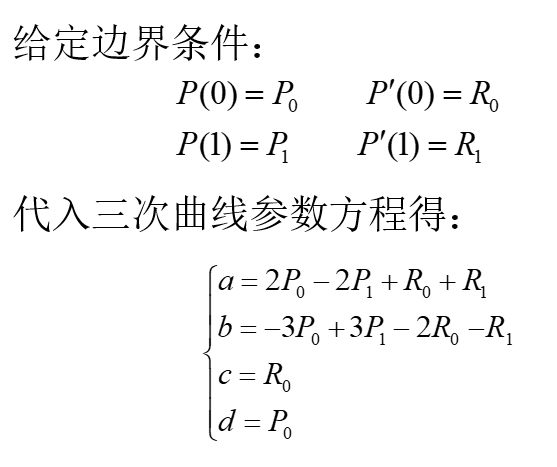Bezier曲线$P_i$是控制点
有n个控制点，那么曲线的阶数就是n-1，计算量巨大
Bezier曲线的性质

端点性质  曲线过两端点且与端点相切
对称性:  若保持n次Bezier曲线的顶点的位置不变，而把次序颠倒，则曲线保持不变
凸包性： 伯恩斯坦多项式各项之和为1。这意味着Bezier曲线各点均落在特征多边形顶点构成的凸包之中
几何不变性：曲线的形状仅与特征多边形个顶点的相对位置有关，而与坐标的选择无关

B样条曲线这个图是PPT上的，和我下面的有些许不同，但实际是一样的。这个图是我要讲的，下面就逐步来解释

啥是B样条曲线？
整条曲线用一个完整的表达形式，但内在的量是一段一段的，比如一堆的3次曲线拼过去，两条之间满足2次连续
怎么分段呢？每一段的基函数怎么求？
de Boor-Cox递推定义
只要是k阶（k-1次）的B样条基函数，构造一种递推的公式，由0次构造1次，1次构造2次，2次构造3次…依次类推B样条函数定义区间 $u\in[u_{k-1},u_{n+1}]$ 是怎么来的？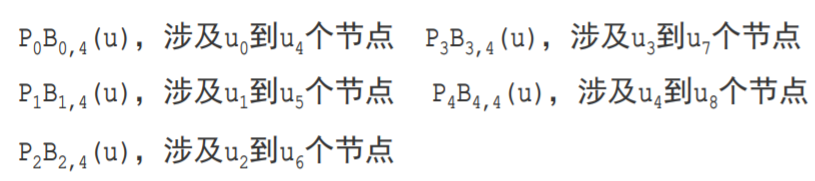B样条曲线的性质

端点性质与连续性
当t=0 或 t=1分别代入三次B样条方程得：
$P(0)=1/3*((p_i+p_{i+2})/2+2p_{i+1})$
$P(1)=1/3*((p_{i+1}+p_{i+3})/2+2p_{i+2})$
三次B样条在连接处的一阶导数、二阶导数都是连续的。‘
局部性：改变一个控制点的位置，最多影响四个曲线段。由此三次B样条具有改变控制点的位置就可以对B样条局部修改曲线
扩展性：增加一个控制点就相应增加一段B样条曲线，而原有曲线不受任何影响

图形变换
平移变换
二维三维比例变换
二维三维旋转变换
二维三维
绕z轴旋转绕x轴旋转绕y轴旋转对称变换错切变换投影变换
正交平行投影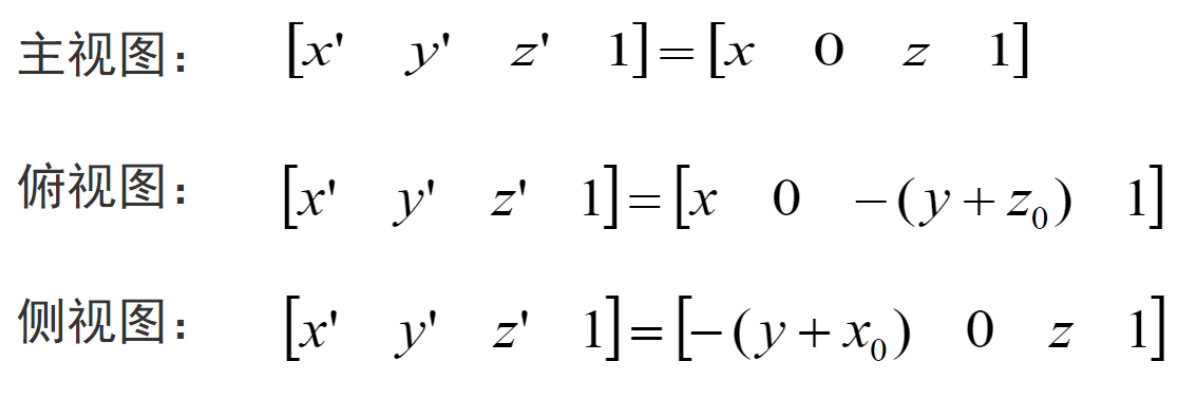斜交投影透视投影展开全文• Sutherland-Hodgman算法 Sutherland-Hodgman算法也叫逐边裁剪法，该算法是萨瑟兰德(I．E．Sutherland)和霍德曼(Hodgman)在1974年提出的。这种算法采用了分割处理、逐边裁剪的方法。计算机图形学
• 采用了分割处理、逐边裁剪的方法。一次用窗口的一条边裁剪多边形，考虑窗口的一条边以及延长线构成的裁剪线该线把平面分成两个部分:可见一侧；不可见一侧。多边形的各条边的两端点S、P。它们与裁剪线的位置关系只有...
• Sutherland-Hodgman算法也叫逐边裁剪法，该算法是萨瑟兰德(I．E．Sutherland)和霍德曼(Hodgman)在1974年提出的。这种算法采用了分割处理、逐边裁剪的方法。一，基本思想: 一次用窗口的一条边裁剪多边形。 考虑窗口的...
• 逐边裁剪算法 基本思想： 该算法依次用窗口的四条边框直线对多边形进行分步裁剪。先用一条边框直线对整个多边形进行裁剪，得到一个或若干个新的多边形；再用第二条边框直线对这些新产生的多边形进行裁剪。依次类
• 使用Java编写的一款简单的直线、多边形的生成和裁剪软件，符合计算机图形...采用Java的drawLine()方法模拟OpenGL的绘点过程，从另一个方面验证并实现了中点bresenham算法、逐边裁剪算法等。有需要的同学可以下载参考！
• 实现圆弧生成的中点算法，实现多边形生成的扫描线算法,实现一般连通区域的基于扫描线的种子填充算法，实现直线段的基本裁剪算法Cohen-Shutherland算法和中点算法，实现多边形图形的逐边裁剪算法，实现二维图形的基本...
• 　Sutherland-Hodgman算法也叫逐边裁剪法，该算法是萨瑟兰德(I．E．Sutherland)和霍德曼(Hodgman)在1974年提出的。这种算法采用了分割处理、逐边裁剪的方法。 　一、Sutherland-Hodgeman多边形裁剪算法思想： 　...
• （两种线段裁剪算法和H-S多边形逐边裁剪算法）多边形裁剪算法的动画演示要求先画出一个封闭的多边形，再画矩形的裁剪窗口，然后选择裁剪按钮（或命令），按下“上边裁剪”按钮（或执行“上边裁剪”命令），多边形...
• 　Sutherland-Hodgman算法也叫逐边裁剪法，该算法是萨瑟兰德(I．E．Sutherland)和霍德曼(Hodgman)在1974年提出的。这种算法采用了分割处理、逐边裁剪的方法。 　一、Sutherland-Hodgeman多边形裁剪算法思想： 　...
• （两种线段裁剪算法和H-S多边形逐边裁剪算法）多边形裁剪算法的动画演示要求先画出一个封闭的多边形，再画矩形的裁剪窗口，然后选择裁剪按钮（或命令），按下“上边裁剪”按钮（或执行“上边裁剪”命令），多边形...
• （两种线段裁剪算法和H-S多边形逐边裁剪算法）多边形裁剪算法的动画演示要求先画出一个封闭的多边形，再画矩形的裁剪窗口，然后选择裁剪按钮（或命令），按下“上边裁剪”按钮（或执行“上边裁剪”命令），多边形...
•  Sutherland-Hodgman算法也叫逐边裁剪法，该算法是萨瑟兰德(I．E．Sutherland)和霍德曼(Hodgman)在1974年提出的。这种算法采用了分割处理、逐边裁剪的方法。 一，基本思想:  一次用窗口的一条边裁剪多边形。  ...
• 任务描述 ...Southerland-Hodgman 多边形裁减算法的基本思想就是逐边进行裁减;首先将多边形对于巨型窗口的裁剪分解为对窗口四边形所在直线的裁剪,其次,将多边形对一条直线的裁剪分解为各条边对直线的裁剪
• (4) 线段裁剪算法可以为点判断法、cohn-Sutherland算法等；其它算法不限； 8、 几何变换 (1) 图形变换包括对二维图形进行平移、旋转、缩放、对称等变换，以及对三维图形进行平移、旋转、缩放等变换； (2) 平移、...
• 二、逐边裁剪算法 三、视图窗口的扩缩变换 四、视图窗口裁剪图形与扩缩变换的程序设计 第三节 动画程序设计 一、改变颜色模拟运动 二、用异或方式模拟运动 三、用显示擦除模拟运动 第四节 二维图形矩阵变换 一、点...
• 4.8 AABB与场景相交 2.4.9 更精确的碰撞检测 2.4.10 使用碰撞阈值 2.5 基本的路径规划 附录2.1 实时处理的演示 第3章 高级游戏系统剖析Ⅲ：软件设计与应用编程 3.1 应用的种类 3.1.1 插件 3.1.2 前端 3.1.3 工具...
• 4.8 AABB与场景相交 2.4.9 更精确的碰撞检测 2.4.10 使用碰撞阈值 2.5 基本的路径规划 附录2.1 实时处理的演示 第3章 高级游戏系统剖析Ⅲ：软件设计与应用编程 3.1 应用的种类 3.1.1 插件 3.1.2 前端 3.1.3 工具...
• 演示文档可以页播放或是自动播放，还有多种页面切换方式供您选择，如“展开”、“卷入”、“溶解”等。单个对象也可专门设置演示方式。在解说时您可以用鼠标模拟的“粉笔”在屏幕上补充和评点，并可以利用工具或...